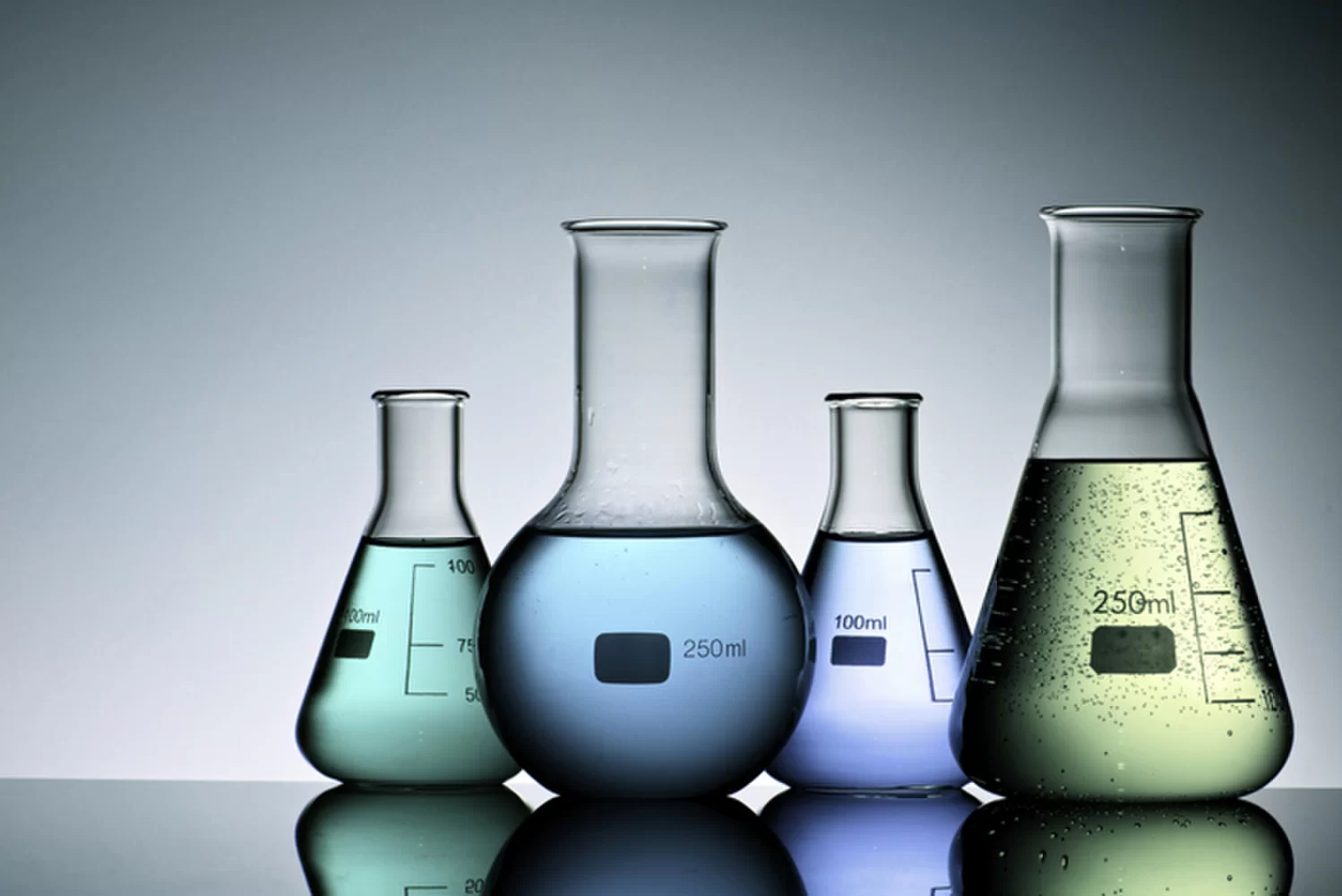# Quiz Over Behavior Of Liquids And Gases

100 Questions | Attempts: 296SettingsThere are three main states of matter namely, solids, liquids, and gasses. In this quiz, we look deeply into the volume of liquids and gasses and their behaviors in different conditions. All the best as you test and advance your knowledge.

• 1.
What is a major difference between a gas and a liquid?
• A.

A liquid is far less compressible than a gas.

• B.

A gas is far less compressible than a liquid.

• C.

A liquid is more combustible than a gas.

• D.

A gas is more combustible than a liquid.

• 2.
What is the lifting force of a liquid called?
• A.

Laminar

• B.

Interface

• C.

Buoyance

• D.

Dynamic pressure

• 3.
Which best describes immiscible liquids?
• A.

They mix well together

• B.

One liquid will float on the other

• C.

They will become an entrained solution

• D.

They will repel each other and react violently

• 4.
Which unit of measurement would indicate velocity of flow?
• A.

Feet per second

• B.

Gallons per minute

• C.

Cubic feet per second

• D.

Cubic gallons per minute

• 5.
Which physical factor affects the behavior of liquids in both rest and motion?
• A.

Friction, applied forces, and gravity

• B.

Friction, inertia, and atmospheric pressure

• C.

Gravity, atmospheric pressure, and inertia

• D.

Gravity, atmospheric pressure, and applied forces

• 6.
What determines the intensity of the excess pressure in a water-hammer wave?
• A.

The size of the pipe

• B.

The specific gravity of the fuel

• C.

The change in gallons per minute

• D.

The amount of extinguished velocity

• 7.
Which type of measurement may be used to express pressure?
• A.

Kilograms

• B.

Pounds

• C.

Tons

• D.

Pounds per square inch

• 8.
The standard measure for atmospheric pressure is equal to how many pounds per square inch?
• A.

13.1 psi

• B.

14.7 psi

• C.

15.2 psi

• D.

17.0 psi

• 9.
What type of pressure reading is also referred to as overpressure?
• A.

Zero pressure

• B.

Gauge pressure

• C.

Absolute pressure

• D.

Atmospheric pressure

• 10.
What term is used to describe the total pressure acting as a gas?
• A.

Overpressure

• B.

Gauge pressure

• C.

Absolute pressure

• D.

Atmospheric pressure

• 11.
Which temperature scale is commonly used for scientific measurements?
• A.

Kelvin scale

• B.

Rankine scale

• C.

Reaumur scale

• D.

Fahrenheit scale

• 12.
Which gas law declares that cooling a gas decreases its volume?
• A.

Boyle’s law

• B.

Charles’ law

• C.

Dalton’s law

• D.

Gay-Lussac’s law

• 13.
Two or more atoms combined is called a
• A.

A compound

• B.

An element

• C.

A molecule

• D.

A mixture

• 14.
According to the electron theory, atoms are composed of
• A.

Protons, neutrons, and electrons

• B.

Neutrons, elements, and protons

• C.

Electrons, molecules, and elements

• D.

Electrons, neutrons, and matter

• 15.
How do the charges of protons and electrons differ?
• A.

Protons have positive charges, and electrons have neutral charges

• B.

Protons have neutral charges, and electrons have positive charges

• C.

Protons have negative charges, and electrons have positive charges

• D.

Protons have positive charges, and electrons have negative charges

• 16.
The uniform movement of electrons in a specific direction is called
• A.

Electrical potential

• B.

Free electrons

• C.

Electric current

• D.

Ionization

• 17.
Which shell of the valence electrons determines whether a material is a good or a poor conductor of electricity?
• A.

The outermost shell

• B.

The middle shell

• C.

The second shell

• D.

The first shell

• 18.
Which statement best describes a positive ion?
• A.

An atom that lost a proton and has more electrons

• B.

An atom that lost an electron and has more protons

• C.

An atom that has an equal number of electrons and protons

• D.

An atom that cannot throw off any protons

• 19.
What effect of current causes an electric motor to produce mechanical energy?
• A.

Heat

• B.

Magnetism

• C.

Chemical

• D.

Reactance

• 20.
What is the unit of measurement of electrical power?
• A.

Watts

• B.

Volts

• C.

Amps

• D.

Ohms

• 21.
A 3-hoursepower motor consumes how much power?
• A.

755 W

• B.

1,534 W

• C.

2,023 W

• D.

2,238 W

• 22.
A magnet that is magnetized by induction from an external source is called?
• A.

A natural magnet

• B.

An electromagnet

• C.

An artificial magnet

• D.

An electromechanical magnet

• 23.
When do the magnetic lines of force in a magnet cross?
• A.

When the north pole of a magnet is placed near the north pole of another magnet.

• B.

When the south pole of a magnet is placed near the north pole of another magnet.

• C.

When a permanent magnet and a temporary magnet are put together.

• D.

Magnetic lines of force never cross each other.

• 24.
What are the two most common methods of producing voltage?
• A.

Magnetic and heat

• B.

Chemical and heat

• C.

Chemical and mechanical

• D.

Magnetic and mechanical

• 25.
What type of current flow is the result of voltages produced by batteries and thermocouples?
• A.

Open current

• B.

Direct current

• C.

Latent current

• D.

Alternating current

## Related TopicsBack to top
×

Wait!
Here's an interesting quiz for you.